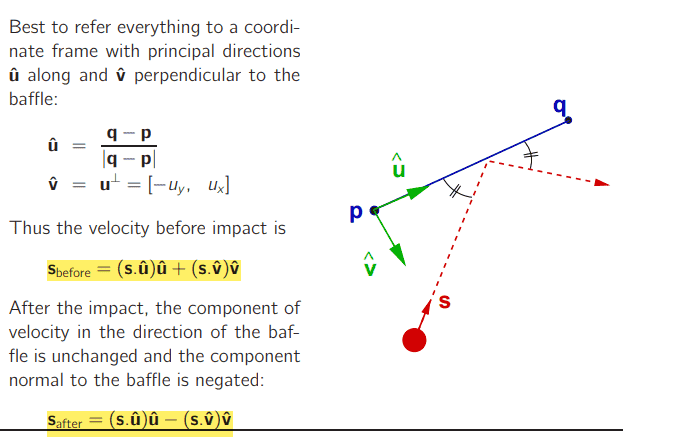# Pinball with velocity vector

• MHB
• WMDhamnekar

#### WMDhamnekar

MHB
Hi,

A pinball moving in a plane with velocity s bounces (in a purely elastic impact) from a baffle whose endpoints are p and q. What is the velocity vector after the bounce?

I don't understand how to answer this question? Any math help, hint or even correct answer will be accepted?

Use vectors addition and elastic collision concept that velocity along the baffle will remain unchanged and velocity perpendicular to baffle will get reversed.

You can always set up a coordinate with P as origin and Q= (0, 1). The velocity vector of this object can be written $(v_x, v_y)$ in that coordinate system. After an elastic collision with PQ, it's velocity vector is $(-v_x, v_y)$.

You can always set up a coordinate with P as origin and Q= (0, 1). The velocity vector of this object can be written $(v_x, v_y)$ in that coordinate system. After an elastic collision with PQ, it's velocity vector is $(-v_x, v_y)$.
Hi,

Author has given the following answer to this question. Would you tell me how does the highlighted terms relate to velocity before and after the bounce?A vector $u = u_x + u_y$ you can write a vector as a sum of its components.
$(s. \hat{u} )$ represents the magnitude of the component of vector s along baffle and if you multiply by unit vector $\hat{u}$ you get vector component of s along with the baffle similarly $(s. \hat{v})$ represents the magnitude of the component of vector s normal to baffle and again if you multiply by unit vector $\hat{v}$ you will get vector component of s normal to baffle.
For reflected ray normal gets reversed so the normal vector is expressed with the negative sign there.